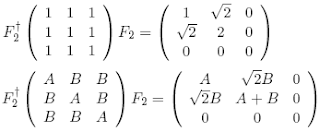occasional meanderings in physics' brave new world

Thursday, October 09, 2008

CKM Rules II

Recall that the tribimaximal mixing matrix (ie. the MNS) is expressed in the form $F_{3}F_{2}$ for two Fourier operators, where $F_3$ naturally diagonalises $3 \times 3$ 1-circulants and the two dimensional operator $F_{2}$ acts on the democratic matrix and other special circulantsto reduce the circulant to two dimensions. A similar 2-circulant yields the element $B - A$ in the $C_{33}$ entry. Note that the approximate CKM sum of two such 1-circulants also gives a reduced matrix, by linearity. Only CP violation and the 2-circulant contribution keeps the quark mixing solidly in three dimensions.

Observe that if 2 is really -1, then the reduced matrix is of the form $\sigma_{Z} + i \sigma_{X}$, a Pauli q-number. So MUB algebras really do suggest the use of modular arithmetic.

Update: I should also mention that there is a factor of $\frac{2}{3}$ missing here; the 3 from the Fourier normalisation.